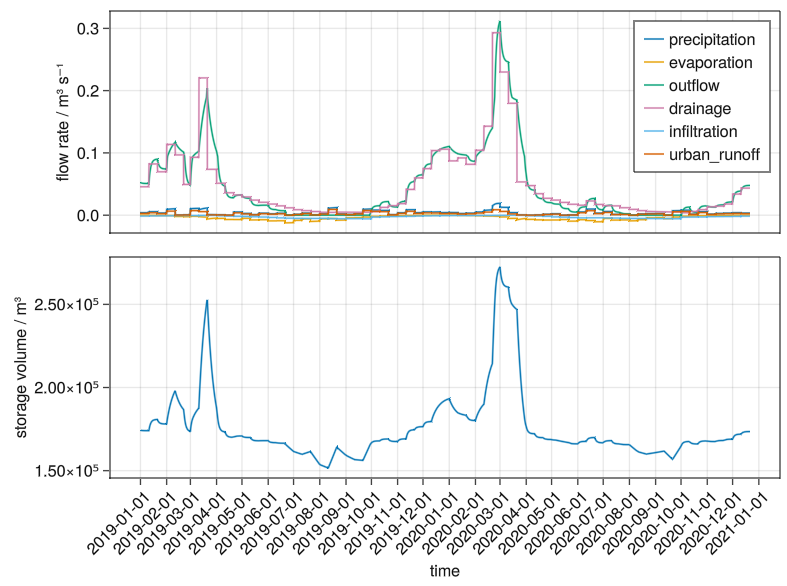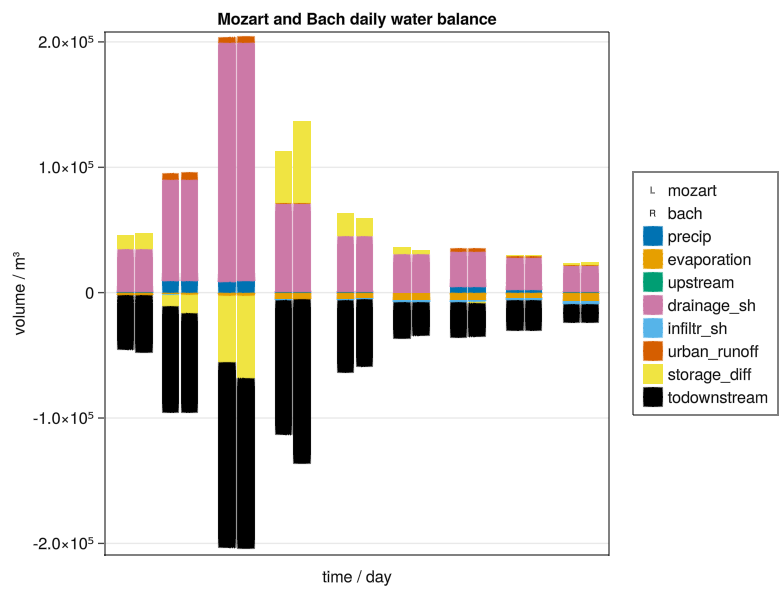# Ribasim

Ribasim is a water resources model, designed to be the replacement of the regional surface water modules Mozart and SIMRES in the Netherlands Hydrological Instrument (NHI). Ribasim is a work in progress, it is a prototype that demonstrates all essential functionalities. Further development of the prototype in a software release is planned in 2022 and 2023.

Ribasim is written in the Julia programming language and is built on top of the SciML: Open Source Software for Scientific Machine Learning libraries, notably DifferentialEquations.jl.

For most users the latest release is recommended, it can be downloaded here:

The nightly builds contain the latest developments and can be found below. It is important to either use the release or nightly for all components.

Currently only Windows builds for ribasim_cli.zip are available.

flowchart TB
modeler([Modeler]):::user

api["Ribasim Python\n[python]"]:::system
modeler-->|prepare model|api

ribasim["Ribasim\n[julia]"]:::system
modeler-->|start|ribasim

subgraph qgisBoundary[QGIS]
QGIS[QGIS Application]:::system_ext
qgisPlugin["Ribasim QGIS plugin\n[python]"]:::system
QGIS-->qgisPlugin
end
modeler-->|prepare model|qgisBoundary

model[("input model data\n[toml + geopackage + arrow]")]:::system
ribasim-->|simulate|model

output[("simulation results\n[arrow]")]:::system
ribasim-->|write|output

class qgisBoundary boundary

%% class definitions for C4 model
classDef default stroke-width:1px,stroke:white,color:white
classDef system fill:#1168bd
classDef user fill:#08427b
classDef system_ext fill:#999999
classDef boundary fill:transparent,stroke-dasharray:5 5,stroke:black,color:black


System overview of Ribasim

# 2 Status

The initial focus is on being able to reproduce the Mozart regional surface water reservoir results. Each component is defined by a set of symbolic equations, and can be connected to each other. From this a simplified system of equations is generated automatically. We use solvers with adaptive time stepping from DifferentialEquations.jl to get results.Example timeseries of a single basin, the Hupselse Beek, with the input and output fluxes on the top, and the storage volume (the state) below.Example bar plot of the daily waterbalance for the Hupselse Beek, comparing results of Mozart (left) and Ribasim (right).

# 3 Introduction

## 3.1 Water balance equations

The water balance equation for a drainage basin can be defined by a first-order ordinary differential equation (ODE), where the change of the storage $$S$$ over time is determined by the inflow fluxes minus the outflow fluxes.

$\frac{\mathrm{d}S}{\mathrm{d}t} = Q_{in} - Q_{out}$

We can split out the fluxes into separate terms, such as precipitation $$P$$, evapotranspiration $$ET$$ and runoff $$R$$. For now other fluxes are combined into $$Q_{rest}$$. If we define all fluxes entering our reservoir as positive, and those leaving the system as negative, all fluxes can be summed up.

$\frac{\mathrm{d}S}{\mathrm{d}t} = R + P + ET + Q_{rest}$

## 3.2 Time

The water balance equation can be applied on many timescales; years, weeks, days or hours. Depending on the application and available data any of these can be the best choice. In Ribasim, we make use of DifferentialEquations.jl and its ODE solvers. Many of these solvers are based on adaptive time stepping, which means the solver will decide how large the time steps can be depending on the state of the system.

The forcing, like precipitation, is generally provided as a time series. Ribasim is set up to support unevenly spaced timeseries. The solver will stop on timestamps where new forcing values are available, so they can be loaded as the new value.

Ribasim is essentially a continuous model, rather than daily or hourly. If you want to use hourly forcing, you only need to make sure that your forcing data contains hourly updates. The output frequency can be configured independently. To be able to write a closed water balance, we accumulate the fluxes. This way any variations in between timesteps are also included, and we can output in m³ rather than m³s⁻¹.

## 3.3 Space

The water balance equation can be applied on different spatial scales. Besides modelling a single lumped watershed, it allows you to divide the area into a network of connected representative elementary watersheds (REWs) . At this scale global water balance laws can be formulated by means of integration of point-scale conservation equations over control volumes. Such an approach makes Ribasim a semi-distributed model. In this document we typically use the term “basin” to refer to the REW. (In Mozart the spatial unit was called Local Surface Water (LSW)). Each basin has an associated polygon, and the set of basins is connected to each other as described by a graph, which we call the network. Below is a representation of both on the map.

The network is described as graph. Flow can be bi-directional, and the graph does not have to be acyclic.

graph LR;
A["basin A"] --- B["basin B"];
A --- C["basin C"];
B --- D["basin D"];
C --- D;


Internally a directed graph is used. The direction is defined to be the positive flow direction, and is generally set in the dominant flow direction. The basins are the nodes of the network graph. Basin states and properties such storage volume and wetted area are associated with the nodes (A, B, C, D), as are most forcing data such as precipitation, evaporation, or water demand. Basin connection properties and interbasin flows are associated with the edges (the lines between A, B, C, and D) instead.

Multiple basins may exist within the same spatial polygon, representing different aspects of the surface water system (perennial ditches, ephemeral ditches, or even surface ponding). Figure 1, Figure 2, Figure 3 show the 25.0 m rasterized primary, secondary, and tertiary surface waters as identified by BRT TOP10NL in the Hupsel basin (as defined in the Mozart LSW’s). These systems may represented in multiple ways.

As a single basin (A) containing all surface water, discharging to its downstream basin to the west (B):

graph LR;
A["basin A"] --> B["basin B"];


Such a system may be capable of representing discharge, but it cannot represent residence times or differences in solute concentrations: within a single basin, drop of water is mixed instantaneously. Instead, we may the group primary (P), secondary (S), and tertiary (T) surface waters. Then T may flow into S, S into P, and P discharges to the downstream basin (B.)

graph LR;
T["basin T"] --> S["basin S"];
S --> P["basin P"];
P --> B["basin B"];


As each (sub)basin has its own volume, low throughput (high volume, low discharge, long residence time) and high throughput (low volume, high discharge, short residence time) systems can be represented in a lumped manner; of course, more detail requires more parameters.